# Bogolyubov chain of equations

(diff) ← Older revision | Latest revision (diff) | Newer revision → (diff)

BBGKY-equations, Bogolyubov–Born–Green–Kirkwood–Yvon equations

A chain of equations (hierarchy) for the one-particle, two-particle, etc., distribution functions of a classical statistical system. The functions are defined as follows: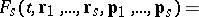(1)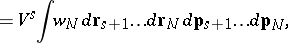whereis the volume of the system and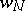is the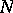-particle normalized distribution function which satisfies the Liouville equation: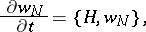(2)

where the brackets are the Poisson brackets and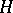is the Hamiltonian of the system. In the thermodynamical limit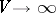,, the chain of equations has the form of an equation for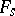with a specific "link" with the function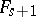of a higher rank: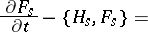(3)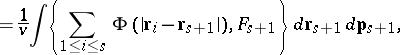where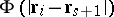is the potential of the interaction between the-th and the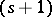-th particles, whileis the Hamiltonian of the-particle system.

Under conditions of thermodynamic equilibrium when the momentum distribution of each particle is the Maxwell distribution, one considers the-particle coordinate distribution functions of particles, defined by relations of type (1) in terms of an-particle function(4)

where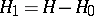, and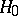is the sum of the kinetic energies of the particles of the system, while the configuration integralis defined by the normalization condition (4). The chain of equations for these functions has the form(5)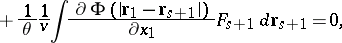whereis the potential energy of the interaction betweenparticles of the system.

The distribution functions, in particularand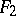, may be used to express all the specific characteristics of statistical systems. The principal difficulties involved in the study of the functions (3) or (5) are caused by the problems of closing the system (decoupling the chain of equations) and solving the closed system under special boundary conditions for the functions. This study is essential for physical systems of various kinds and is most advanced for the case of short-range interaction, when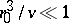, whereis the effective radius of interaction between the particles, and for the case of long-range interaction, when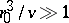, in particular for systems in which electrostatic (Coulomb) forces are operative. In the time-dependent theory this leads directly to the kinetic Boltzmann equation for the single-particle function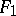or to the Vlasov kinetic equation, and in the equilibrium theory to the virial decomposition for the thermodynamic potential or to specific Coulomb corrections.

In considering quantum-statistical systems, the BBGKY-hierarchy is constructed for-particle statistical quantum operators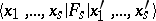which are traces over the variables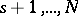of the particles of the general-particle operator — the density matrix. The form of these equations is similar to that of (3), in which the classical Poisson brackets have been replaced by quantum brackets.

How to Cite This Entry:
Bogolyubov chain of equations. Encyclopedia of Mathematics. URL: http://encyclopediaofmath.org/index.php?title=Bogolyubov_chain_of_equations&oldid=16782
This article was adapted from an original article by I.A. Kvasnikov (originator), which appeared in Encyclopedia of Mathematics - ISBN 1402006098. See original article